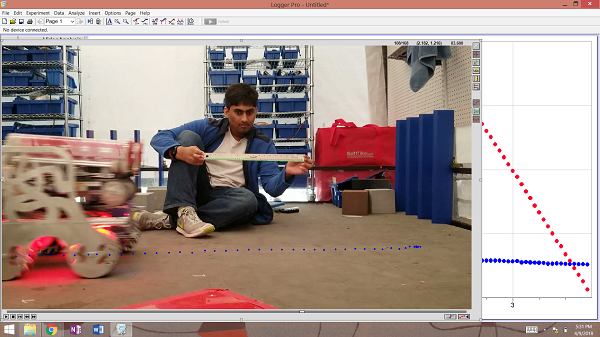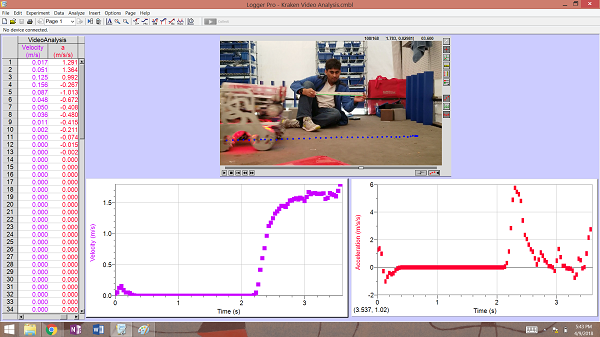# Robot Video Analysis

Tags: design and think
Personhours: 3
Robot Video Analysis By Charlotte

### Task: Determine the acceleration and max velocity experimentally

To find the acceleration and maximum velocity of our robot we decided to use a method we have learned in our physics class at school: video analysis with Logger Pro. The procedure is like so: Take a video of the robot head on with a still camera. In the video, in the same frame of movement as the robot, hold a known measuring device (ruler/meter stick). Insert the video into Logger Pro, use the ruler tool to set the distance of the measuring device you used to its length and use the point tool to place a point on the same part of the robot (like the front wheel) for every frame. You can see the collection of points in the image below:Logger Pro automatically makes a displacement and velocity graph for X and Y. We are interested in the X direction unless your robot is flying. To make an acceleration graph, create a new calculated column that takes the derivative of the X velocity graph. Both graphs are shown below:Finally it is time to analyze our data. To find max velocity: use the stats tool on the point where the velocity is done increasing and has become constant. To find the acceleration: in this case the acceleration is not constant, so we are looking to find the average acceleration in the beginning when the robot is speeding up from rest by using the stats tool again on the portion of the acceleration graph that occurs at the same time as the velocity initially increases, right before it becomes constant. These were our results:

Max Velocity: 1.67 m/s | Average Acceleration: 2.58 m/s^2

We did this video analysis in order to better understand our robot. We will use this information when we are making code changes to the robot in these last days before Worlds.

### Next Steps:

We have made determined many aspects of our robot experientially, the coefficient of friction of our internal lift, etc. In the future we will use these skills to find out more abour our robot.

Date | April 10, 2018